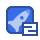# Understanding Machine Learning && Solution Manual.zip27收藏

Machine learning is one of the fastest growing areas of computer science, with far-reaching applications. The aim of this textbook is to introduce machine learning, and the algorithmic paradigms it offers, in a principled way. The book provides a theoretical account of the fundamentals underlying machine learning and the mathematical derivations that transform these principles into practical algorithms. Following a presentation of the basics, the book covers a wide array of central topics unaddressed by previous textbooks. These include a discussion of the computational complexity of learning and the concepts of convexity and stability; important algorithmic paradigms including stochastic gradient descent, neural networks, and structured output learning; and emerging theoretical concepts such as the PAC-Bayes approach and compression-based bounds. Designed for advanced undergraduates or beginning graduates, the text makes the fundamentals and algorithms of machine learning accessible to students and non-expert readers in statistics, computer science, mathematics and engineering.

...展开详情

• 4
资源
• 1
粉丝
•等级Understanding Machine Learning && Solution Manual.zip 32积分/C币 立即下载
1/0